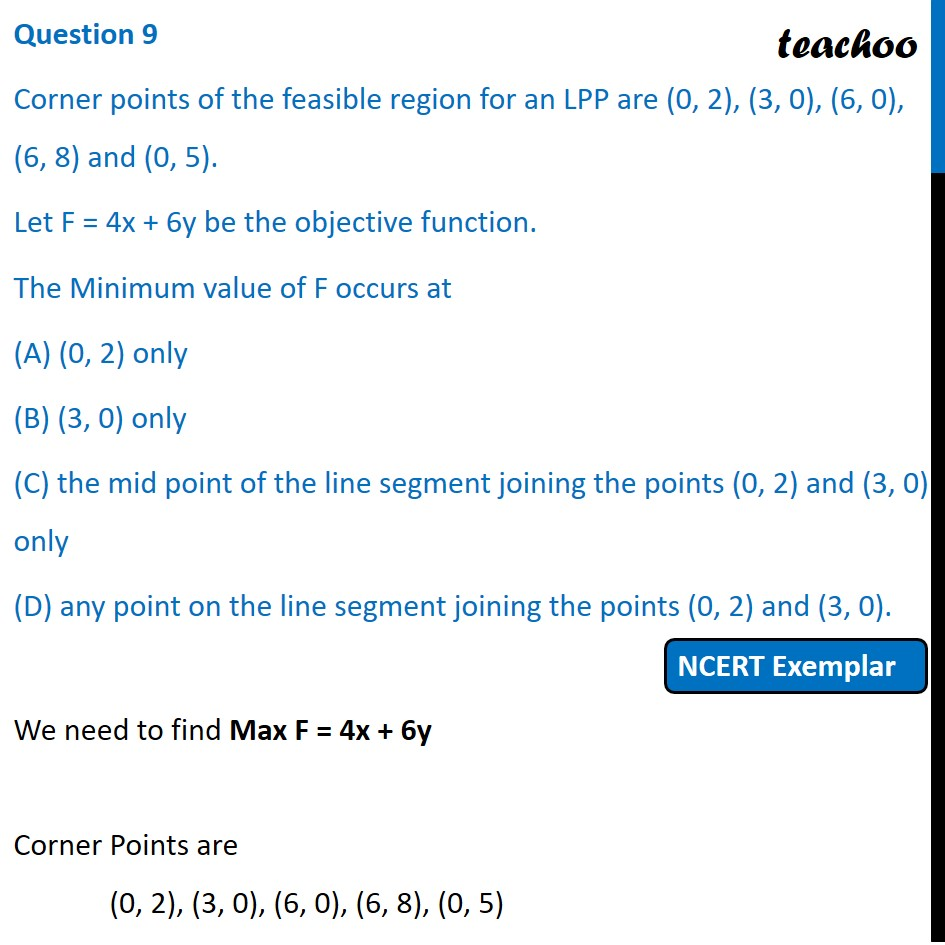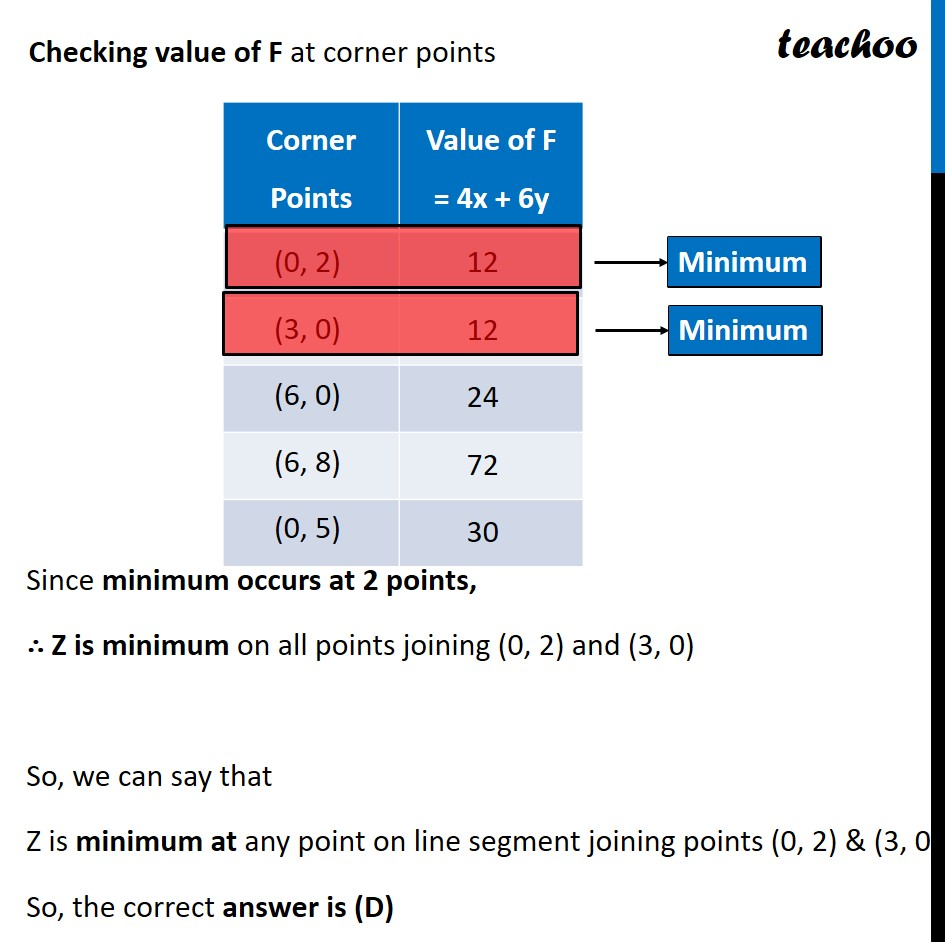NCERT Exemplar - MCQs

Chapter 12 Class 12 Linear Programming
Serial order wise

## (D) any point on the line segment joining the points (0, 2) and (3, 0).

This question is similar to Question 38 Choice 2 - Sample Paper 2021 Class 12 - Linear ProgrammingLearn in your speed, with individual attention - Teachoo Maths 1-on-1 Class

### Transcript

Question 9 Corner points of the feasible region for an LPP are (0, 2), (3, 0), (6, 0), (6, 8) and (0, 5). Let F = 4x + 6y be the objective function. The Minimum value of F occurs at (A) (0, 2) only (B) (3, 0) only (C) the mid point of the line segment joining the points (0, 2) and (3, 0) only (D) any point on the line segment joining the points (0, 2) and (3, 0). We need to find Max F = 4x + 6y Corner Points are (0, 2), (3, 0), (6, 0), (6, 8), (0, 5) Checking value of F at corner points Since minimum occurs at 2 points, ∴ Z is minimum on all points joining (0, 2) and (3, 0) So, we can say that Z is minimum at any point on line segment joining points (0, 2) & (3, 0). So, the correct answer is (D)